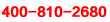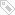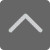# 一年级上册数学期末试卷-小学数学一年级上册第四单元测试题

2019-01-09 22:52:40 　来源：网络整理

一年级上册数学期末试卷-小学数学一年级上册第四单元测试题一年级的你是不是有在学校好好听课？下面就是小编为大家带来的一年级上册数学期末试卷-小学数学一年级上册第四单元测试题，希望可以帮助到大家。

一、直接写得数。20分

36÷6= 24÷6= 9÷3= 12÷4= 5×3=

30+6= 12÷6= 15÷5= 36÷6= 6×6=

3×4= 5×5= 25÷5= 5×6= 20÷4=

4×3= 15÷3= 3×4= 2×6= 24-6=

二、填空。16分(每空1分)

1、把10支铅笔平均分成5份，每份是( )支。列算式是：

2、25÷5=( )，读作( )除以( )，被除数是( )，除数是( )，商是( )。

3、18÷6=( )想：( )六十八，商是( )

4、把口诀补充完整，二九( )，根据这个口诀写出两道除法算式

5、12÷4=3，表示把12平均分成( )份，每份是( )。

三、选正确答案，把序号填在( )里8分

1、5个6相加写成算式是( ) A: 6+5 B: 6×5 C: 5+6

2、把18个苹果平均分在3个盘子里，每盘6个，写成除法算式是( ) 　　A: 18÷3=6 B: 18÷6=3 C: 3×6=18

3、6÷6( )6-6 A: > B: < C: =

4、3×( )<17，括号里最大可以填( ) A: 4 B: 6 C: 5

四、在○里填上“>”、“<”或“=”。9分

3×5○15÷3 12÷6○3×4 20÷4○5 3×6○30÷6

6×4○3×6 18÷3○2×3 3×4○12÷2 6÷6○36

五、在○里填上“+”、“-”或“×”。8分

23○3=20 2○2=4 24○4=6

5○5=0 5○5=25 　　5○4=20

2○5=10 3○3=9 4○4=1

小编推荐：

以上就是小编特意为大家整理的一年级上册数学期末试卷-小学数学一年级上册第四单元测试题希望对有需要的同学提供帮助，大家如果在学习中有什么疑问，欢迎拨打爱智康免费电话：!那里有专业的老师为大家解答。

### 2019年精品学习包：

•### 小学语|数|英

历年试卷、北京30所校练习题、名著|重点校巡礼|等100讲视频、纸质书籍领取

•### 初中九大学科

历年真题、期末|期中|志愿填报|重点校巡礼|等100讲视频、纸质书籍领取

•### 高中九大学科

历年真题、期末|期中|志愿填报|重点校巡礼|等100讲视频、纸质书籍领取

•### 更新中

学习包内容持续更新中......标签：• 全国课程在线咨询
• 咨询热线：4000-121-121
• 扫描注册有礼

• 让学习更有效